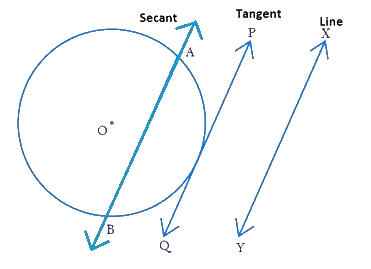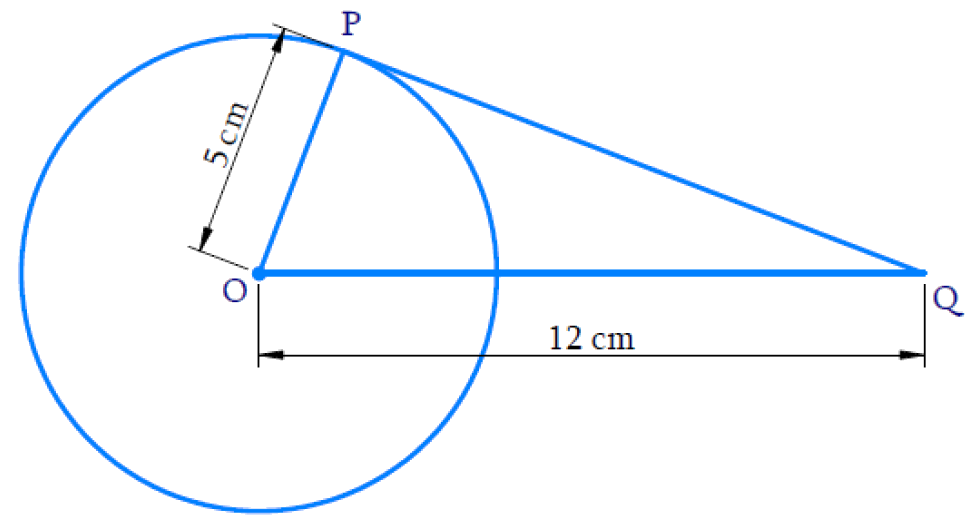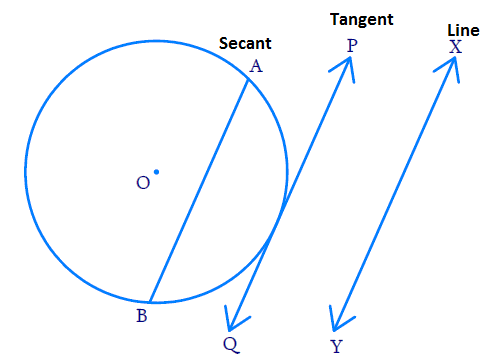# NCERT Solutions For Class 10 Maths Chapter 10 Exercise 10.1

Go back to  'Circles'

## Chapter 10 Ex.10.1 Question 1

How many tangents can a circle have?

### Solution

What is Unknown?

Number of tangents a circle can have

Reasoning:

A tangent to a circle is a line that intersects the circle at only one point. On every point on the circle, one tangent can be drawn.

Steps:

As per the above reasoning, a circle can have infinitely many tangents.

## Chapter 10 Ex.10.1 Question 2

Fill in the blanks:

(i) A tangent to a circle intersects it in _________ point (s).

(ii) A line intersecting a circle in two points is called a ____________.

(iii) A circle can have _________ parallel tangents at the most.

(iv) The common point of a tangent to a circle and the circle is called _________ .

### Solution

Steps:

(i) A tangent to a circle intersects it in       One         point (s).

Reasoning:

A tangent to a circle is a line that intersects the circle at only one point.

(ii) A line intersecting a circle in two points is called a    Secant   .

Reasoning:

Secant is a line that intersects the circle in two points.(iii) A circle can have    Two     parallel tangents at the most.

Reasoning:

Tangent at any point of a circle is perpendicular to the radius through the point of contact. Extended radius is a diameter which has two end points and hence two tangents which are parallel to themselves and perpendicular to the diameter.

Center $$O,$$ diameter $$AB,\text{ tangents}\, PQ, RS$$ and, $${PQ}\; \| \;{RS}$$

$$A$$ and $$B$$ are called as point of contact.

(iv) The common point of a tangent to a circle and the circle is called    Point of contact     .

Reasoning:

A tangent to a circle is a line that intersects the circle at only one point and that point is called as point of contact.

## Chapter 10 Ex.10.1 Question 3

A tangent $$PQ$$ at a point $$P$$ of a circle of radius $$\text{5 cm}$$ meets a line through the center $$O$$ at a point $$Q$$ so that $$OQ = \text{12 cm.}$$ Length $$PQ$$ is:

(A) $$\text{12 cm}$$

(B) $$\text{13 cm}$$

(C) $$\text{8.5 cm}$$

(D) $$\sqrt{119} \,\rm{cm.}$$

### Solution

What is Known?

Radius $$OP = \text{5 cm}$$

$$OQ = \text{12 cm}$$What is Unknown?

Length of the tangent $$PQ$$

Reasoning:

$$\Delta {OPQ}$$ is a right-angle triangle according to Theorem $$10.1 :$$ The tangent at any point of a circle is perpendicular to the radius through the point of contact.

Steps:

By Pythagoras theorem

\begin{align} {OQ} ^ { 2 } & = {O P} ^ { 2 } + {P Q} ^ { 2 } \\ 12 ^ { 2 } & = 5 ^ { 2 } + {P Q} ^ { 2 } \\ 144 & = 25 + {P Q} ^ { 2 } \\ {P Q} ^ { 2 } & = 119 \\ {P Q} & = \sqrt { 119 } \rm {\;cm } \end{align}

## Chapter 10 Ex.10.1 Question 4

Draw a circle and two lines parallel to a given line such that one is a tangent and the other, a secant to the circle.

### Solution

What is Known?

(i) To draw a circle

(ii) Draw one tangent and one secant to the circle parallel  to the given line.

What is Unknown?

To draw a circle as per known details.

Steps:$$XY$$ is the given line.

$$AB$$ is the secant parallel to $$XY,$$ $${AB}\parallel {XY}$$

$$AQ$$ is the tangent parallel to $$XY,$$ $${PQ}\parallel {XY}$$